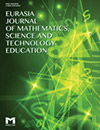# Duality of Mathematical Thinking When Making Sense of Simple Word Problems : Theoretical EssayPolotskaia, E., Savard, A., & Freiman, V. (2015). Duality of Mathematical Thinking When Making Sense of Simple Word Problems : Theoretical Essay. Eurasia Journal of Mathematics, Science & Technology Education, 11(1), 213–223. https://doi.org/10.12973/eurasia.2015.1325a

Abstract: 7.1.This essay proposes a reflection on the learning difficulties and teaching approaches associated with arithmetic word problem solving. We question the development of word problem solving skills in the early grades of elementary school. We are trying to revive the discussion because first, the knowledge in question—reversibility of arithmetic operations and flexibility of mathematical thinking—are the key elements in elementary mathematics, and second, because we hope to create a shift in the understanding of this knowledge development in students. Using the folk tale ―The Three Little Pigs‖ as a metaphor, we analyze difficulties students experience while learning to solve word problems involving addition and subtraction. We formulate a hypothesis about cognitive duality of word problem-solving. This hypothesis explains some well-known learning difficulties and suggests teaching principles which would help to avoid developmental obstacles and pitfalls within the teaching/learning process.